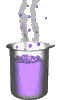Science class online
Chemistry and physics lessons

# Mechanics

Free online mechanics lesson for elementary school,  middle school and high school.Science class Chemistry Electricity Optics Mechanics Mechanics lessons Gravity - The solar system - motions of celestial bodies - Physical interactions - Effects of gravity - Weight of an object - Difference between weight and mass - Relationship between weight and mass  Energy - Energy basics - Potential energy - Kinetic energy - Mechanical energy - Translational Kinetic Energy - kinetic energy mass and speed

 _____________________________________________________   _____________________________________________________

## Gravity

### Relationship between mass and weight

Mass can be measured with a balance whereas weight can be measured with a dynamometer.
Several measures of masses and weights of different objects can be used to compare them and establish an eventual relationship between them.

The following table indicates in its columns the mass, the weight and the ratio between these two quantities (W / m)

 Object Object 1 Object 2 Object 3 Object 4 Mass m (in kg) 0,1 0,2 0,5 1 Weight W (In N) 1 2 5 10 Ratio ( W : m ) 10 10 10 10

The  ratio W:m remains constant ( its value is 10 ), such a result shows that weight is proportional to mass: on Earth, the weight of an object correspond to roughly ten times its mass.

The coefficient of proportionality between weight and mass is denoted by g and called gravitational field strenght.
The gravitational field strenght is expressed in newton per kilogram ( N/kg ) and its value on Earth is nearly 10 N/kg. More accurate measures would have led to a result of 9,81 N/kg
The proportionality between weight and mass lead to the following relationship:

W = m x g

With:

W: Weight (in newton)
m: mass (in kilogram)
g: gravitationnal field strenght (in newton per kilogram)
______________________________________

______________________________________

____________________________________

____________________________________